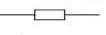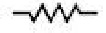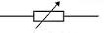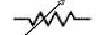What do resistors do?

Resistors limit the current flow in circuits. In most typical hobby circuits resistors are used in conjunction with LED's. The table below shows the two main types of resistors and their different symbols used in circuits. Resistors can be connected in a circuit with either end positive or negative.Fixed resistor EUFixed resistor USVariable resistor EUVariable resistor US

There are other types of resistors such as, thermistors and light dependent resistors (LDR) but for basic hoppy circuits we don't need to cover these just yet.

Pretty much all electronic circuits require resistors to make them work properly so it is important to find out about the different types of resistor available, and to be able to choose the correct resistor value, in Ω, KΩ , or MΩ , for a your application.

There are three types of fixed value resistors, carbon film, metal film and wire wound resistors.

Carbon film resistors are cheap and easily available, with values within ±10% or ±5% of their marked value.

Metal film resistors are made in a similar way to carbon film resistors, but are made with more accurately, this is to within ±2% or ±1% of their nominal value.

Wire wound resistors are made extremely accurately and are for use in multimeters, oscilloscopes and other measuring equipment. Some types of wirewound resistors can pass large currents without overheating and these are used in power supplies and other high current circuits.

Resistor colour codes

Resistors are colour banded to help determine their values. For help determining the value of a resistor please use our 4 band resistor calculator.

Power Rating

When current flows through a resistor the electrical energy is converted into heat. It is very important to select the right power rating of your resistor so to avoid overheating and burring the resistor out. In order to calculate the correct power rating the Joule's Law formula is used. This is further explained and the formula for the calculation is on our Ohms Law Calculator page.

The power output is calculated by P=VI, where P is power, V is volts and I is current. Consider the following,

Volts = 5, current = 100ma

5x100mA = 500mW equivalent to 0.5W

The most common application for resistors in hobby circuits is to limit the current flow for LED's The best way to select the correct value and power rating of your resistor is to use our LED resistor calculator.